Download Presentation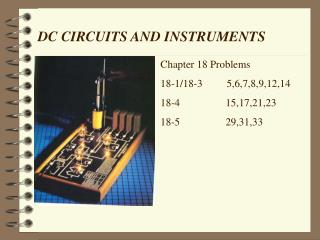DC CIRCUITS AND INSTRUMENTS

# DC CIRCUITS AND INSTRUMENTS - PowerPoint PPT Presentation

DC CIRCUITS AND INSTRUMENTS. Chapter 18 Problems 18-1/18-3 5,6,7,8,9,12,14 18-4 15,17,21,23 18-5 29,31,33. DC CIRCUITS AND INSTRUMENTS. OBJECTIVESI am the owner, or an agent authorized to act on behalf of the owner, of the copyrighted work described.
Download Presentation## DC CIRCUITS AND INSTRUMENTS

An Image/Link below is provided (as is) to download presentation

Download Policy: Content on the Website is provided to you AS IS for your information and personal use and may not be sold / licensed / shared on other websites without getting consent from its author.While downloading, if for some reason you are not able to download a presentation, the publisher may have deleted the file from their server.

- - - - - - - - - - - - - - - - - - - - - - - - - - E N D - - - - - - - - - - - - - - - - - - - - - - - - - -
Presentation Transcript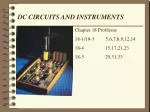DC CIRCUITS AND INSTRUMENTS

Chapter 18 Problems

18-1/18-3 5,6,7,8,9,12,14

18-4 15,17,21,23

18-5 29,31,33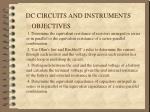### DC CIRCUITS AND INSTRUMENTS

OBJECTIVES

1. Determine the equivalent resistance of resistors arranged in series or in parallel or the equivalent resistance of a series-parallel combination.

2. Use Ohm's law and Kirchhoff’ s rules to determine the current through each resistor and the voltage drop across each resistor in a single loop or multiloop dc circuit.

3. Distinguish between the emf and the terminal voltage of a battery and calculate the terminal voltage given the emf internal resistance of the battery and external resistance in the circuit.

4. Determine the equivalent capacitance of capacitors arranged in series or in parallel or the equivalent capacitance of a series-parallel combination.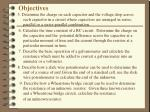Objectives

5. Determine the charge on each capacitor and the voltage drop across each capacitor in a circuit where capacitors are arranged in series, parallel or a series-parallel combination.

6. Calculate the time constant of a RC circuit. Determine the charge on the capacitor and the potential difference across the capacitor at a particular moment of time and the current through the resistor at a particular moment in time.

7. Describe the basic operation of a galvanometer and calculate the resistance which must be added to convert a galvanometer into an ammeter or a voltmeter.

8. Describe how a slide wire potentiometer can be used to determine the emf of a source of emf.Given the emf of a standard cell, use the slide wire potentiometer to calculate the emf of the unknown.

9. Describe how a Wheatstone bridge circuit can be used to determine the resistance of an unknown resistor. Given three known resistors and a Wheatstone bridge circuit, calculate the resistance

of an unknown resistor.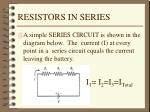RESISTORS IN SERIES
• A simple SERIES CIRCUIT is shown in the diagram below. The current (I) at every point in a series circuit equals the current leaving the battery.

I1= I2=I3=ITotal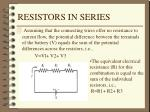RESISTORS IN SERIES
• Assuming that the connecting wires offer no resistance to current flow, the potential difference between the terminals of the battery (V) equals the sum of the potential differences across the resistors, i.e.,

V=Vl+ V2+ V3

• The equivalent electrical resistance (R) for this combination is equal to the sum of the individual resistors, i.e.,
• R=R1+ R2+ R3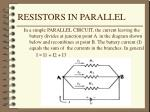RESISTORS IN PARALLEL

In a simple PARALLEL CIRCUIT, the current leaving the battery divides at junction point A in the diagram shown below and recombines at point B. The battery current (I) equals the sum of the currents in the branches. In general

I = I1 + I2 + I3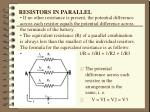RESISTORS IN PARALLEL
• If no other resistance is present, the potential difference across each resistor equals the potential difference across the terminals of the battery.
• The equivalent resistance (R) of a parallel combination is always less than the smallest of the individual resistors. The formula for the equivalent resistance is as follows:
• 1/R = 1/RI + 1/R2 + 1/R3
• The potential difference across each resistor in the arrangement is the same, i. e.
• V = VI = V2 = V3RESISTORS IN PARALLEL

In a simple PARALLEL CIRCUIT, the current leaving the battery divides at junction point A in the diagram shown below and recombines at point B. The battery current (I) equals the sum of the currents in the branches. In general

I = I1 + I2 + I3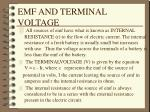EMF AND TERMINAL VOLTAGE
• All sources of emf have what is known as INTERNAL RESISTANCE (r) to the flow of electric current. The internal resistance of a fresh battery is usually small but increases with use. Thus the voltage across the terminals of a battery is less than the emf of the battery.
• The TERMINALVOLTAGE (V) is given by the equation V =  - Ir, where  represents the emf of the source of

potential in volts, I the current leaving the source of emf in amperes and r the internal resistance in ohms.

• The internal resistance of the source of emf is always considered to be in a series with the external resistance present in the electric circuit.KIRCHHOFF'S RULES
• KIRCHHOFF'S RULES are used in conjunction with Ohm's law in solving problems involving complex circuits:
• KIRCHHOFF'S FIRST RULE or JUNCTION RULE: The sum of all currents entering any junction point equals the sum of all currents leaving the junction point. This rule is based on the law of conservation of electric charge.
• KIRCHHOFF'S SECOND RULE or LOOP RULE: The algebraic sum of all the gains and losses of potential around any closed path must equal zero. This law is based on the law of conservation of energy.SUGGESTIONS FOR USING KIRCHHOFF'S LAWS

1. Place a (+) sign next the long line of the battery symbol and a (-) sign next to the short line. Start choosing a direction for conventional current flow ( flow of positive charge )

If you choose the wrong direction for the flow of current in a particular branch, your final answer for the current in that branch will be negative. The negative answer indicates that the current actually flows in the opposite direction.

ISUGGESTIONS FOR USING KIRCHHOFF'S LAWS
• 2. Assign a direction to the circuit in each independent branch of the circuit. Place a positive sign on the side of each resistor where the current enters and a negative sign on the side where the current exits, e.g.; This indicates that a drop in potential occurs as the current passes through the resistor .SUGGESTIONS FOR USING KIRCHHOFF'S LAWS
• Notice how the directions of the currents are labeled in each branch of the circuitSUGGESTIONS FOR USING KIRCHHOFF'S LAWS
• 3. Select a JUNCTION POINT and apply the junction rule, e.g., at point A in the diagram:

The junction rule may be applied at more than one junction point. In general, apply the junction rule to enough junctions so that each branch current appears in at least one equation.SUGGESTIONS FOR USING KIRCHHOFF'S LAWS
• 4. Apply Kirchhoff’s loop rule by first taking note whether there is a gain or loss of potential at each resistor and source of emf as you trace the closed loop. Remember that the sum of the gains and losses of potential must add to zero.SUGGESTIONS FOR USING KIRCHHOFF'S LAWS

For example, for the left loop of the sample circuit above start at point B and travel clockwise around the loop. Because the direction chosen for the loop is also the direction assigned for the current, there is a gain in potential across the battery (- to +), but a loss of potential across each resistor (+ to -).SUGGESTIONS FOR USING KIRCHHOFF'S LAWS
• Following the path of the current shown in the diagram and using the loop rule, the following equation can be written:SUGGESTIONS FOR USING KIRCHHOFF'S LAWS

The direction taken around the loop is ARBITRARY. Tracing a counterclockwise path around the circuit starting at B, as shown in the above right diagram, there is gain in potential across each resistor to (- to +) and a drop in potential across the battery (+ to -). The loop equation would then be:SUGGESTIONS FOR USING KIRCHHOFF'S LAWS
• Multiplying both sides of the above equation by - 1 and algebraically rearranging, it can be shown that the two equations are equivalent. Be sure to apply the loop rule to enough closed loops so that each branch current appears in at least one loop equation. Solve for each branch current using standard algebraic methods.

“Solve simultaneous equations”CAPACITORS IN SERIES AND PARALLEL
• A circuit with CAPACITORS IN PARALLEL is shown in the diagram below. According to Kirchhoff ‘s loop rule, the potential difference (V) of the source of emf:
• V = VI = V2 = V3CAPACITORS IN PARALLEL
• The total charge stored on the capacitor plates (Q) equals the amount of charge which left the source of:
• Q = Ql + Q2 + Q3 ( Charge is additive)
• and since Q = CV then
• CV = CV1 + CV2 + CV3
• C= C1+ C2 +C3 (Capacitance is additive)CAPACITORS IN SERIES
• For CAPACITORS IN SERIES, the amount of charge (Q) that leaves the source of emf equals the amount of charge that forms on each capacitor:
• Q = Ql = Q2 = Q3CAPACITORS IN SERIES

From Kirchhoffs loop rule, the potential difference across the source of emf (V) equals the sum of the potential differences across the individual capacitors:Circuits containing resistors and capacitors

An RC CIRCUIT consists of a resistor and a capacitor connected in series to a de power source.When switch 1 (S1), shown in the diagram below, is closed, the current will begin to flow from the source of emf and charge will begin to accumulate on the capacitor. Using Kirchhoff s loop rule it can be shown that